Homework Help Question & Answers

# please answer all parts and show work. 20) The equilibrium constant for e reaction Ni2+ (aa)...

please answer all parts and show work.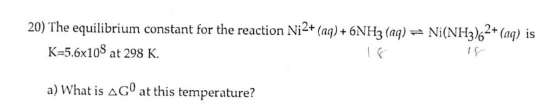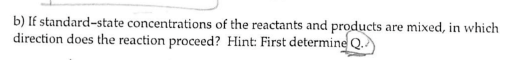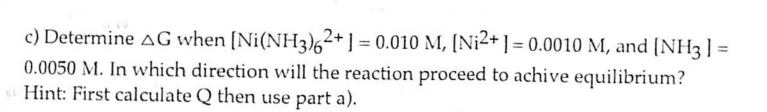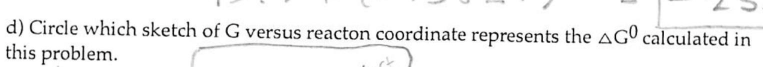20) The equilibrium constant for e reaction Ni2+ (aa) H3 (ag) a Ni(NH3)62+ (ng) is th K-5.6x10s at 298 K. a) What is AG0 at this temperature?

#### Homework Answers

Answer #1

Part A)

Using the following equation we get the dG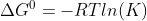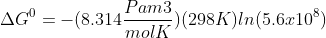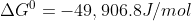Part B

As dG<<0, the process is spontaneous, therefore the reaction at standart conditions of P and T is product favored.

Part C

We need to get K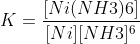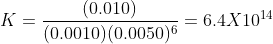Now using the equation  we find dG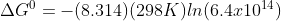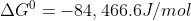Part D

I cant' see the sketch. Please upload a photo with that part of the problem.

Know the answer?
Your Answer:

#### Post as a guest

Your Name:

What's your source?

#### Earn Coin

Coins can be redeemed for fabulous gifts.

Not the answer you're looking for? Ask your own homework help question. Our experts will answer your question WITHIN MINUTES for Free.
Similar Homework Help Questions
• ### value: 10.00 points Check my work Be sure to answer all parts. The equilibrium constant for...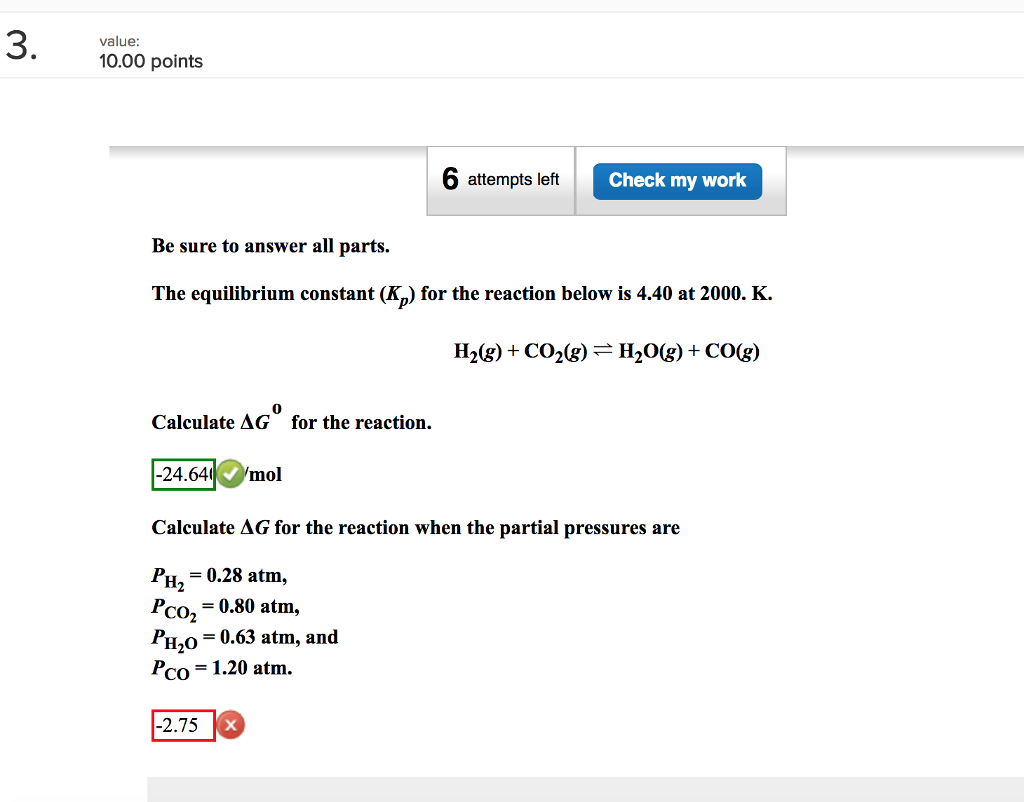value: 10.00 points Check my work Be sure to answer all parts. The equilibrium constant for the reaction below is 4.40 at 2000. K. 0 Calculate AG for the reaction. 24.640/mol Calculate AG for the reaction when the partial pressures are PH2 0.28 atm, PCO2 = 0.80 atm, PHo-0.63 atm, and Pco-1.20 atm. -2.75

• ### The equilibrium constant, K, for a redox reaction is related to the standard potential, Eº, by...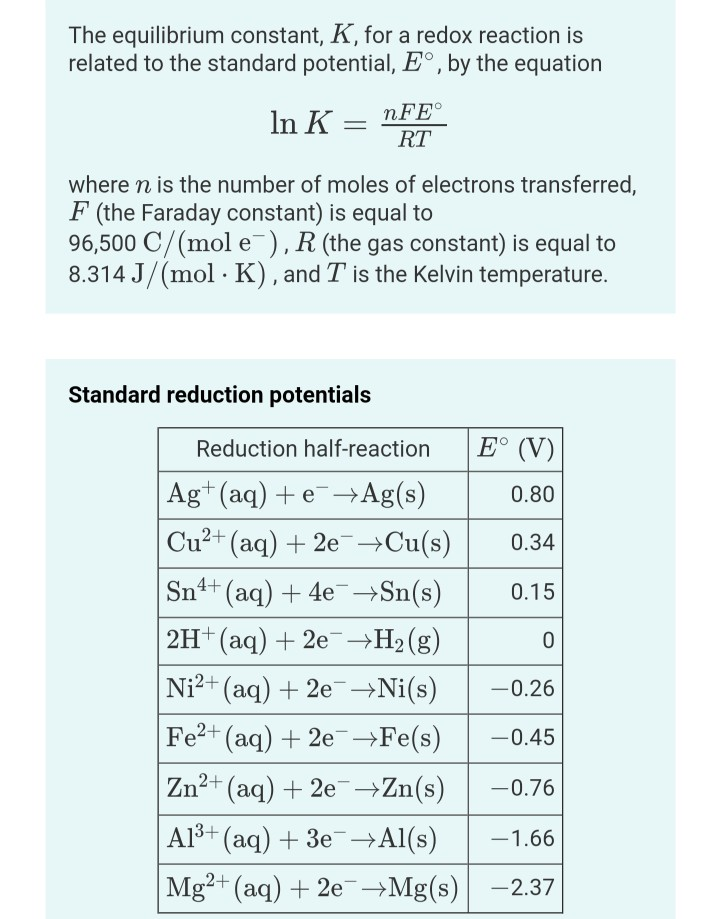The equilibrium constant, K, for a redox reaction is related to the standard potential, Eº, by the equation In K = nFE° RT where n is the number of moles of electrons transferred, F (the Faraday constant) is equal to 96,500 C/(mol e), R (the gas constant) is equal to 8.314 J/(mol · K), and T is the Kelvin temperature. Standard reduction potentials Reduction half-reaction E° (V) Ag+ (aq) + e +Ag(s) 0.80 Cu²+ (aq) + 2e + Cu(s) 0.34...

• ### Please show work step by step and answer. The Temperature Dependence of Chemical Equilibrium For an equilibrium reaction...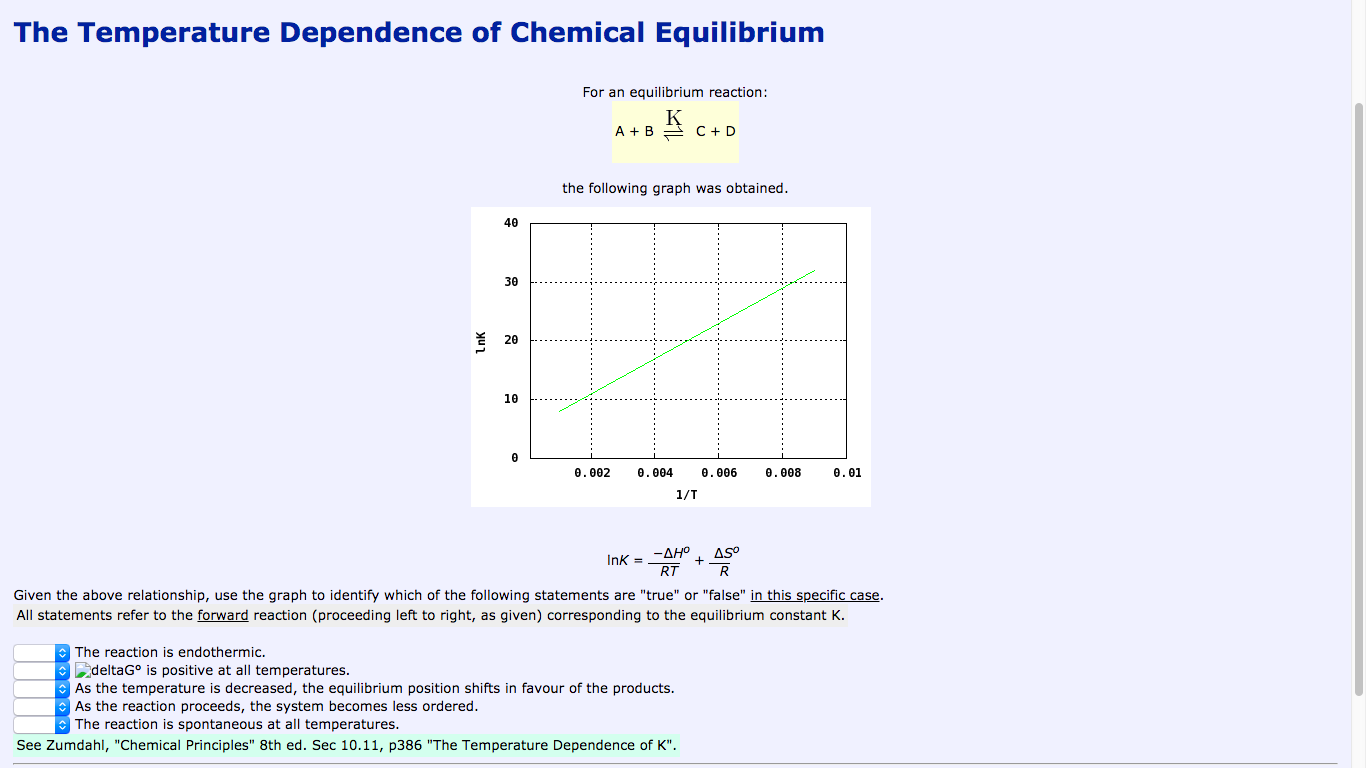Please show work step by step and answer. The Temperature Dependence of Chemical Equilibrium For an equilibrium reaction: - A+B Kc+o the following graph was obtained. 0.002 0.008 0.01 0.004 0.006 1/1 InK = -AHO + 4,50 Given the above relationship, use the graph to identify which of the following statements are "true" or "false" in this specific case. All statements refer to the forward reaction (proceeding left to right, as given) corresponding to the equilibrium constant K. The reaction...

• ### Determine the equilibrium constant at 298 K for the reaction: Zn (s) + Ni2+ (aq) Zn2+...

Determine the equilibrium constant at 298 K for the reaction: Zn (s) + Ni2+ (aq) Zn2+ (aq) + Ni (s) (Hint: Start with the E°) A) (4.1 ± 0.1) x 10 3 B) (1.7 ± 0.1) x 10 17 C) (9.3 ± 0.1) x 10 8 D) (6.4 ± 0.1) x 10 -6 --------------------------------------------------------------------------------------------------------------- Consider the following reaction: CO(g) + H2O(g) CO2(g) + H2(g). Assume ΔH°rxn = 41.0 kJ/mol for all temperatures in this problem. If Kp = 5.10 at...

• ### Cell Potential and Equilibrium Standard reduction potentials The equilibrium constant, K, for a redox reaction is...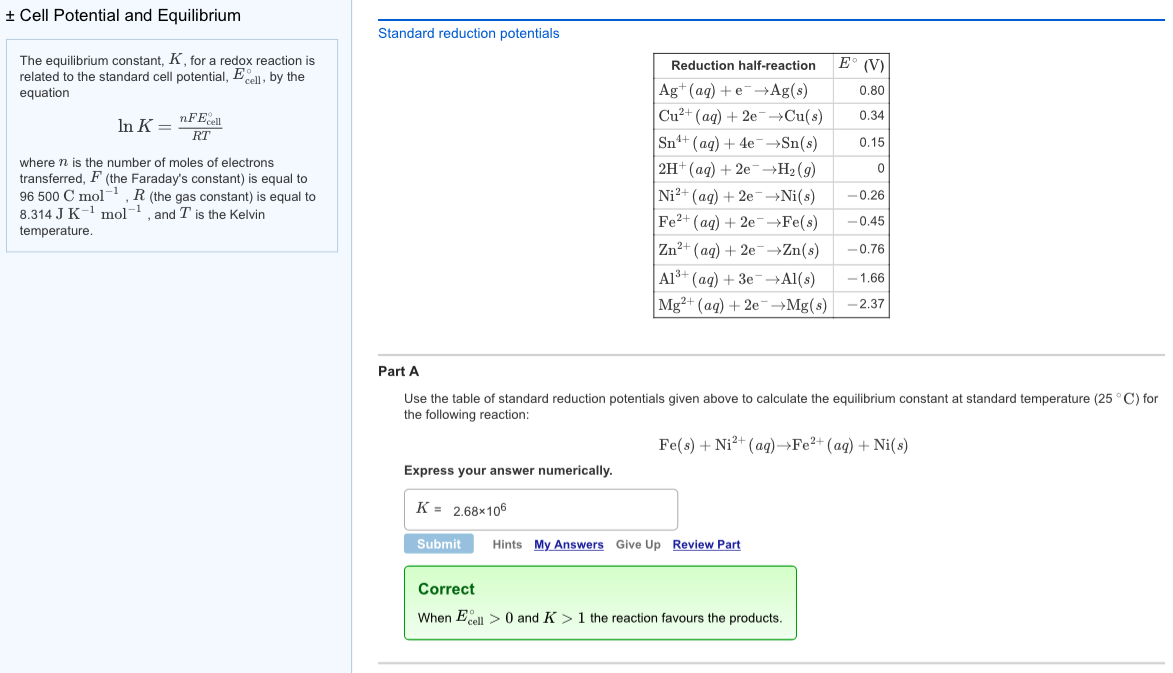Cell Potential and Equilibrium Standard reduction potentials The equilibrium constant, K, for a redox reaction is related to the standard cell potential, Ecel, by the equation Reduction half-reaction (V) Ag+ (aq) + e-→Ag(s) Cu2+ (aq) + 2e-→Cu(s) 0.34 Sn (a) 4e-Sn(s 0.15 2H' (aq) + 2e-→H2 (g) Ni2+ (aq) + 2e-→Ni(s)-0.26 Fe2+ (aq) + 2e-→Fe(s)-0.45 Zn2+ (aq) + 2e-→Zn(s)-0.76 Al3+ (aq) +3e-→Al(s) -1.66 Mg2+ (aq) + 2e-→Mg(s) -2.37 0.80 n FEcell where n is the number of moles of electrons...

• ### Save 3 attempts left Check my work Be sure to answer all parts. The equilibrium constant...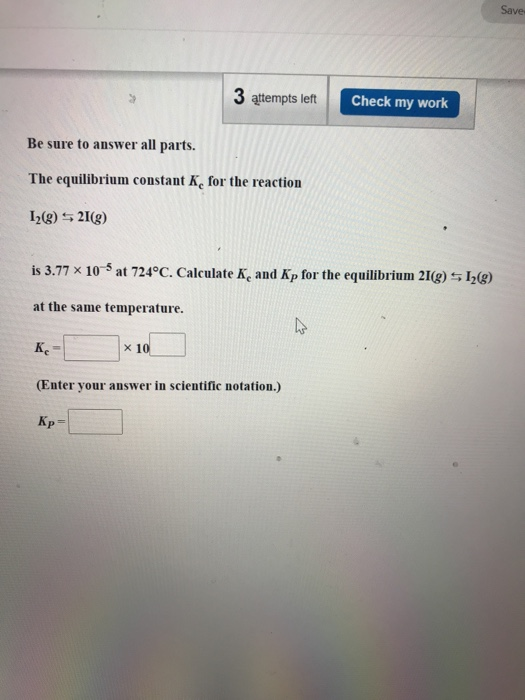Save 3 attempts left Check my work Be sure to answer all parts. The equilibrium constant K, for the reaction 12(g) S 21(9) is 3.77 x 10-5 at 724°C. Calculate K, and Kp for the equilibrium 21(g) 5 12(8) at the same temperature. Kc x 10 (Enter your answer in scientific notation.) Кр

• ### Name SHOW ALL WORK. NO CREDIT UNLESS ALL WORK IS SHOWN. ROUNDING/SIG FIG ERRORS WILL BE...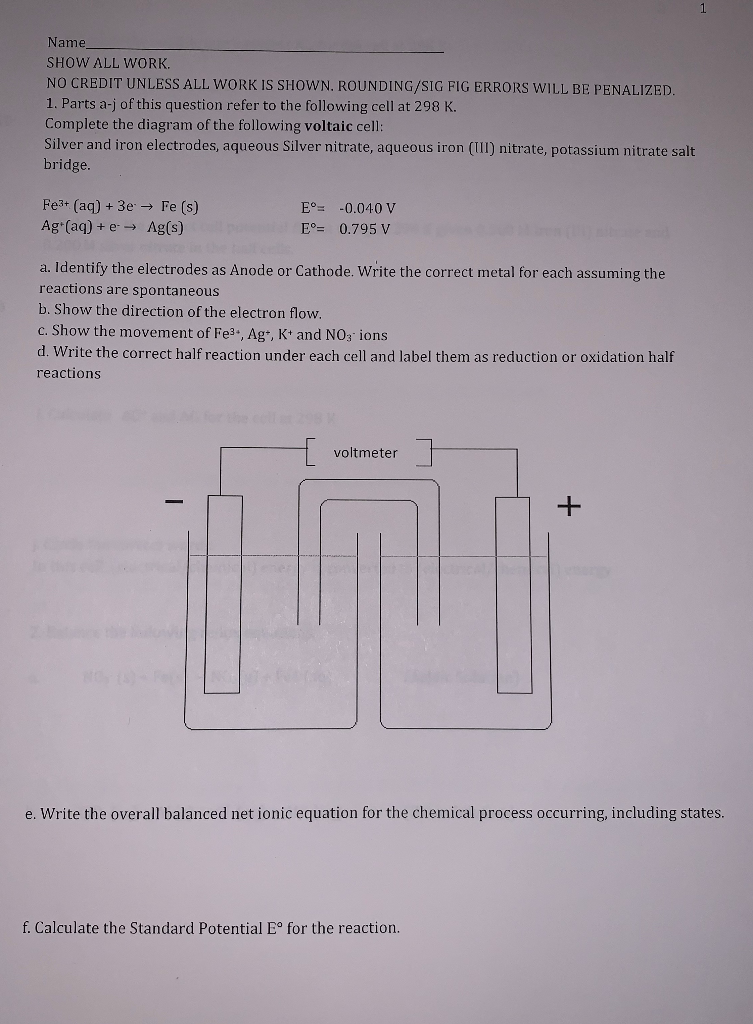Name SHOW ALL WORK. NO CREDIT UNLESS ALL WORK IS SHOWN. ROUNDING/SIG FIG ERRORS WILL BE PENALIZED. 1. Parts a-j of this question refer to the following cell at 298 K. Complete the diagram of the following voltaic cell: Silver and iron electrodes, aqueous Silver nitrate, aqueous iron (UI) nitrate, potassium nitrate salt bridge. Fe3+ (aq) + 3e → Fe (s) Ag (aq) + e - Ag(s) E° -0.040 V Eº= 0.795 V a. Identify the electrodes as Anode or...

• ### The following equilibrium constants were observed for the successive coordination of NH3 with aqueous Ni2+ ion:...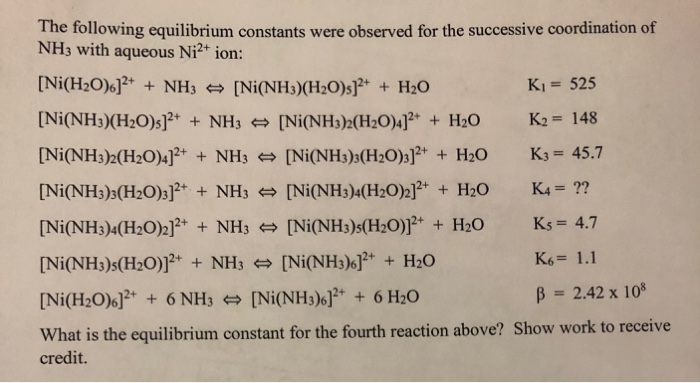The following equilibrium constants were observed for the successive coordination of NH3 with aqueous Ni2+ ion: [Ni(H2O).]2+ + NH3 = [Ni(NH3)(H2O)s]2+ + H2O K1 = 525 [Ni(NH3)(H20)s]2+ + NH3 [Ni(NH3)2(H20)4]2+ + H20 K2 = 148 [Ni(NH3)2(H20)4]2+ + NH3 [Ni(NH3)3(H20)3]2+ + H2O K3 = 45.7 [Ni(NH3)3(H20)3]2+ + NH3 [Ni(NH3)4(H20)2]2+ + H2O K4 = ?? [Ni(NH3)4(H20)2]2+ + NH3 = [Ni(NH3)3(H20)]2+ + H20 Ks = 4.7 [Ni(NH3):(H20)]2+ + NH3 [Ni(NH3).]2+ + H2O K6= 1.1 [Ni(H20)6]2+ + 6 NH3 = [Ni(NH3).]2+ + 6 H2O...

• ### please help with this problem Use th The equilibrium constant, Ke, for the following reaction is...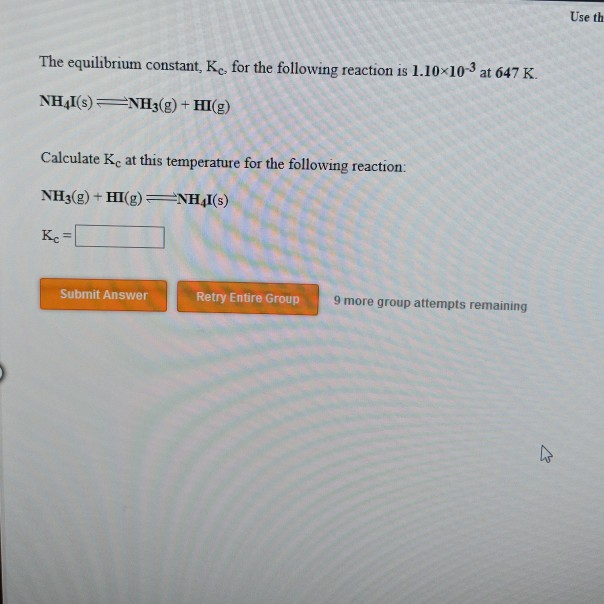please help with this problem Use th The equilibrium constant, Ke, for the following reaction is 1.10x103 at 647 K NHAI(s)NH3g)+HI(g) Calculate Kc at this temperature for the following reaction: NH3(g) + HI(g)4I(s) Кс- Submit Answer Retry Entire Group 9 more group attempts remaining

• ### Please answer BOTH parts to this question. Thanks (1) The equilibrium constant of the reaction 2...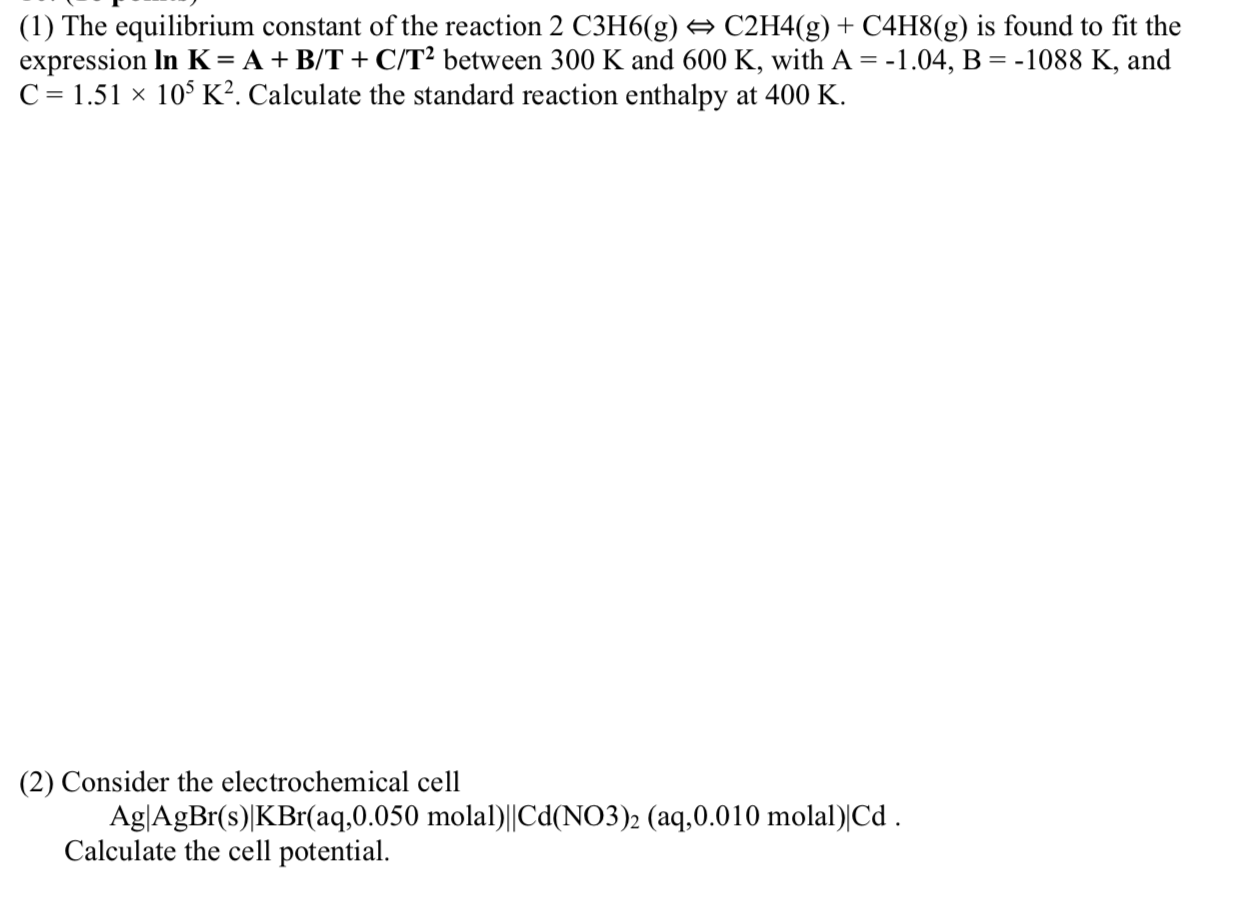Please answer BOTH parts to this question. Thanks (1) The equilibrium constant of the reaction 2 C3H6(g) C2H4(g) + C4H8(g) is found to fit the expression In K= A + B/T + C/T2 between 300 K and 600 K, with A = -1.04, B = -1088 K, and C = 1.51 10% K2. Calculate the standard reaction enthalpy at 400 K. (2) Consider the electrochemical cell Ag|AgBr(s)|KBr(aq,0.050 molal)||Cd(NO3)2 (aq,0.010 molal)|Cd. Calculate the cell potential.